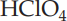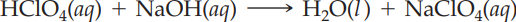×
Get Full Access to Introductory Chemistry - 5 Edition - Chapter 13 - Problem 94p
Get Full Access to Introductory Chemistry - 5 Edition - Chapter 13 - Problem 94p

×

# Solved: A 25.0-mL sample of an unknown HClO4 solution requires 45.3 mL of 0.101 M NaOHISBN: 9780321910295 34

## Solution for problem 94P Chapter 13

Introductory Chemistry | 5th Edition

• Textbook Solutions
• 2901 Step-by-step solutions solved by professors and subject experts
• Get 24/7 help from StudySoup virtual teaching assistantsIntroductory Chemistry | 5th Edition

4 5 1 316 Reviews
19
0
Problem 94P

Problem 94P

A 25.0-mL sample of an unknown HClO4 solution requires 45.3 mL of 0.101 M NaOH for complete neutralization. What was the concentration of the unknown HClO4 solution? The neutralization reaction is:

HClO4(aq) + NaOH(aq) → H2O(l) + NaClO4(aq)

Step-by-Step Solution:

Problem 94P

A 25.0-mL sample of an unknownsolution requires 45.3 mL of 0.101 M NaOH for complete neutralization. What was the concentration of the unknownsolution? The neutralization reaction is:Step by Step Solution

Step 1 of 3

The molarity of the solution is calculated by the following equation;......(1)

Rearrange the formula (1)....(2)

From the given,

Molarity = 0.101M

Volume = 45.3 mL = 0.0453L

Substitute the values in the equation (2)Step 2 of 3

Step 3 of 3

##### ISBN: 9780321910295

The full step-by-step solution to problem: 94P from chapter: 13 was answered by , our top Chemistry solution expert on 05/06/17, 06:45PM. Since the solution to 94P from 13 chapter was answered, more than 1212 students have viewed the full step-by-step answer. This full solution covers the following key subjects: hclo, unknown, solution, naoh, neutralization. This expansive textbook survival guide covers 19 chapters, and 2046 solutions. This textbook survival guide was created for the textbook: Introductory Chemistry, edition: 5. The answer to “A 25.0-mL sample of an unknown HClO4 solution requires 45.3 mL of 0.101 M NaOH for complete neutralization. What was the concentration of the unknown HClO4 solution? The neutralization reaction is:HClO4(aq) + NaOH(aq) ? H2O(l) + NaClO4(aq)” is broken down into a number of easy to follow steps, and 37 words. Introductory Chemistry was written by and is associated to the ISBN: 9780321910295.

Unlock Textbook Solution